• 常见的分类算法有kmeans
千次阅读
2022-07-03 21:23:11

一、什么是聚类

Clustering (聚类)是常见的unsupervised learning (无监督学习)方法。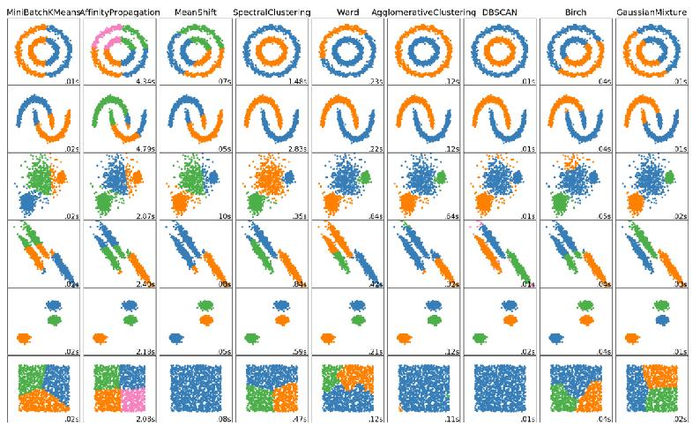聚类的目的就是将大量数据中具有”相似”特征的数据或样本划分到同一个类别中

聚类模型建立在无类别标记的数据上，需要实现的目标只是把相似的样本聚到一起，即只是利用样本数据本身的分布规律

聚类模型会根据数据自身间的”距离”或”相似度”将他们划分成若干组，划分的基本原则就是使组内样本间距离最小化而组间距离最大化

常用的聚类算法如下：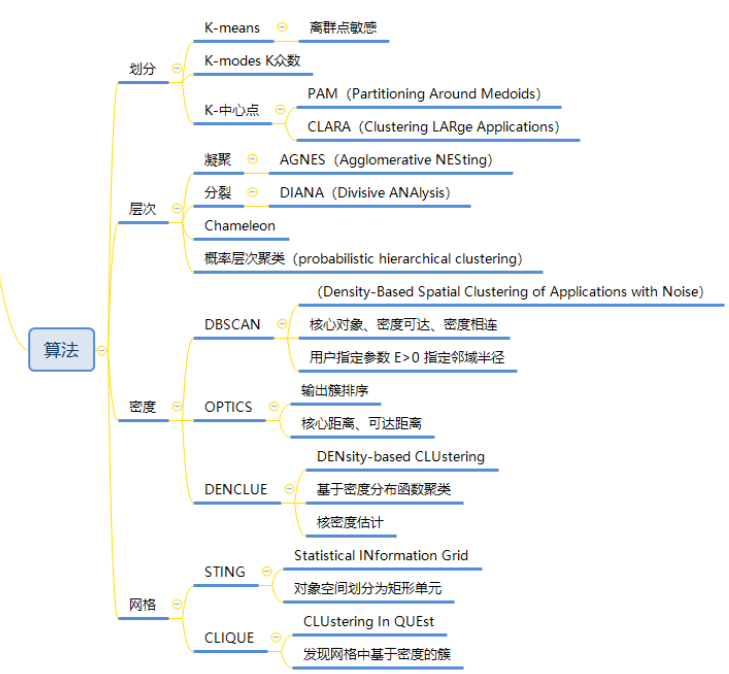二、kmeans算法基本原理

kmeans算法又称k均值算法，是最常用的聚类算法之一，它是基于点与点之间距离的相似度来计算最佳类别归属的方法，需要我们预先确定好聚类的类别数量

kmeans算法使用误差平方和准则函数来评价聚类性能。假设m为各个聚类类别的中心点，p为归属于该类别的样本，则kmeans的目的就是要找到各个点到其归属类别中心距离平方和最小的分类方案&#x机器学习
更多相关内容
• 利用KMeans算法对点云数据进行分类,例如可以提取出点云数据里的房屋、道路等。
• K-Means聚类算法，内附参考文献，亲测可用，性能良好，效果极为明显，完全适用于各种数据维度的数据集分类，欢迎下载使用。
• 大家好呀，我是AI菌，今天和大家分享机器学习里面的简单入门分类算法KMeans、KNN、Meanshift，话不多说我们进入正题！ KMeans k均值聚类算法（k-means clustering algorithm）是一种迭代求解的聚类分析算法，其...

大家好呀，我是AI菌，今天和大家分享机器学习里面的简单入门分类算法：KMeans、KNN、Meanshift，话不多说我们进入正题！

# KMeans

k均值聚类算法（k-means clustering algorithm）是一种迭代求解的聚类分析算法，其步骤是，预将数据分为K组，则随机选取K个对象作为初始的聚类中心，然后计算每个对象与各个种子聚类中心之间的距离，把每个对象分配给距离它最近的聚类中心。聚类中心以及分配给它们的对象就代表一个聚类。每分配一个样本，聚类的聚类中心会根据聚类中现有的对象被重新计算。这个过程将不断重复直到满足某个终止条件。终止条件可以是没有（或最小数目）对象被重新分配给不同的聚类，没有（或最小数目）聚类中心再发生变化，误差平方和局部最小。

KMeans的介绍大家可能看的一知半解，我们用实战运用一下：
我们引入一个已经标注好的样本，并且可视化

import pandas as pd
import numpy as np
X = data.drop(['labels'],axis=1)
y = data.loc[:,'labels']
fig1 = plt.figure()
label0 = plt.scatter(X.loc[:,'V1'][y==0],X.loc[:,'V2'][y==0])
label1 = plt.scatter(X.loc[:,'V1'][y==1],X.loc[:,'V2'][y==1])
label2 = plt.scatter(X.loc[:,'V1'][y==2],X.loc[:,'V2'][y==2])
plt.title("labled data")
plt.xlabel('V1')
plt.ylabel('V2')
plt.legend((label0,label1,label2),('label0','label1','label2'))
plt.show()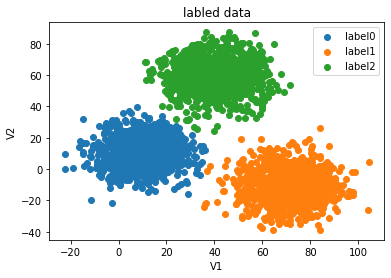运用KMeans模型

from sklearn.cluster import KMeans
KM = KMeans(n_clusters3,random_state=0 #c_clusters为分成若干类，random可理解成每个类随机标签
KM.fit(X)#模型训练
center = KM.cluster_center_#显示训练后的中心点
print(center)


结果：array([[ 40.68362784, 59.71589274],[ 69.92418447, -10.11964119], [ 9.4780459 , 10.686052 ]])
我们把中心点在上图可视化，如下：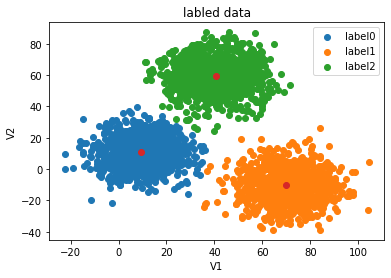模型训练完成后，要进行模型的预测以及预测结果可视化：

y_predict = KM.predict(X)
#visualize the data and results
fig4 = plt.subplot(121)
label0 = plt.scatter(X.loc[:,'V1'][y_predict==0],X.loc[:,'V2'][y_predict==0])
label1 = plt.scatter(X.loc[:,'V1'][y_predict==1],X.loc[:,'V2'][y_predict==1])
label2 = plt.scatter(X.loc[:,'V1'][y_predict==2],X.loc[:,'V2'][y_predict==2])

plt.title("predicted data")
plt.xlabel('V1')
plt.ylabel('V2')
plt.legend((label0,label1,label2),('label0','label1','label2'))
plt.scatter(centers[:,0],centers[:,1])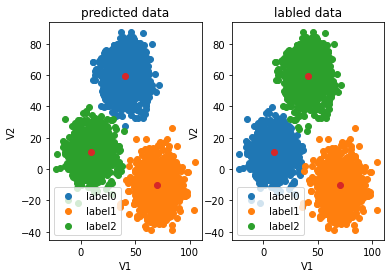对比发现，预测结果和实际结果大致相同，但因为是无监督学习，每个类的标签不一样，所以和实际结果的标签有误，最后进行结果矫正：

y_corrected = []
for i in y_predict:
if i==0:
y_corrected.append(2)
elif i==2:
y_corrected.append(0)
else:
y_corrected.append(1)


最后用y_corrected为标签进行可视化，就完成矫正啦~~而且准确率为0.997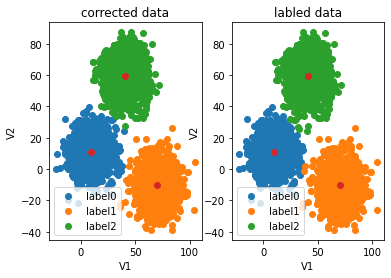# Meanshift

Mean Shift算法的关键操作是通过感兴趣区域内的数据密度变化计算中心点的漂移向量，从而移动中心点进行下一次迭代，直到到达密度最大处（中心点不变）。从每个数据点出发都可以进行该操作，在这个过程，统计出现在感兴趣区域内的数据的次数。该参数将在最后作为分类的依据。
与K-Means算法不一样的是，Mean Shift算法可以自动决定类别的数目。与K-Means算法一样的是，两者都用集合内数据点的均值进行中心点的移动。
下图为MeanShitf的流程图：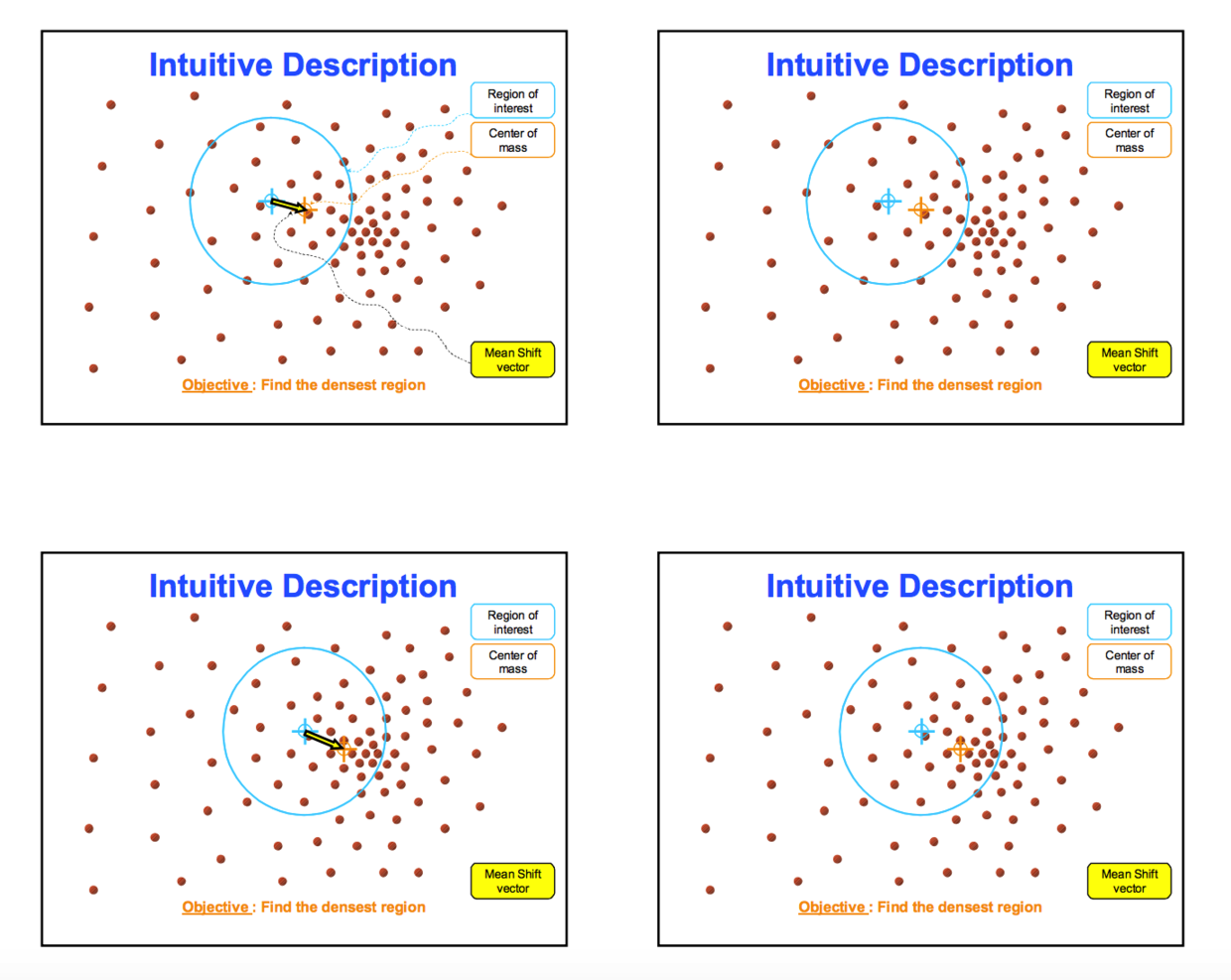实战环节：

from sklearn.cluster import MeanShift,estimate_bandwidth
bw = estimate_bandwidth(X,n_samples=500)#X为原始数据集，n_samples为取若干的点进行估计带宽（半径）
#estimate_bandwidth函数用作于mean-shift算法估计带宽，如果MeanShift函数没有传入bandwidth参数，MeanShift会自动运行estimate_bandwidth
ms = MeanShift(bandwidth=bw)
ms.fit(X)
y_predict_ms = ms.predict(X)
#最后直接进行可视化
fig6 = plt.subplot(121)
label0 = plt.scatter(X.loc[:,'V1'][y_predict_ms==0],X.loc[:,'V2'][y_predict_ms==0])
label1 = plt.scatter(X.loc[:,'V1'][y_predict_ms==1],X.loc[:,'V2'][y_predict_ms==1])
label2 = plt.scatter(X.loc[:,'V1'][y_predict_ms==2],X.loc[:,'V2'][y_predict_ms==2])

plt.title("ms results")
plt.xlabel('V1')
plt.ylabel('V2')
plt.legend((label0,label1,label2),('label0','label1','label2'))
plt.scatter(centers[:,0],centers[:,1])
plt.show()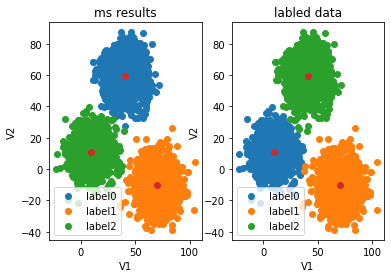因为MeanShift为非监督学习，所以最后标签有可能和原始标签不合，我们要进行结果矫正，方法和KMeans基本相同，就不展示啦~~

knn的话，下期手撸knn源码和大家分享！！

# 拓展

K-means算法是将样本聚类成k个簇（cluster），具体算法描述如下：
1、 随机选取k个聚类质心点（cluster centroids）为2、 重复下面过程直到收敛 :
对于每一个样例i，计算其应该属于的类对于每一个类j，重新计算该类的质心K是我们事先给定的聚类数，Ci代表样例i与k个类中距离最近的那个类，Ci的值是1到k中的一个。质心μi代表我们对属于同一个类的样本中心点的猜测.

展开全文机器学习 数据挖掘
• 用聚类算法kmeans实现图像的分类，自己写详细的注释，包括一份实验报告（报告没啥看的意义）
• MATLAB实现的Kmeans算法，根据输入的初始中心和数据进行分类
• 聚类算法又叫无监督分类，目标是将数据划分为意义的簇，将所有样本按照K个质心进行聚类 质心：一类坐标的平均点 聚类过程：先随机选取K个质心，根据质心生成簇，计算簇的质心，找到新的质心，直到簇与质心不在...

聚类属于无监督学习：训练数据中只有x没有y

聚类算法又叫无监督分类，目标是将数据划分为有意义的簇，将所有样本按照K个质心进行聚类

质心：一类坐标的平均点

聚类过程：先随机选取K个质心，根据质心生成簇，计算簇的质心，找到新的质心，直到簇与质心不在变化，聚类完成

聚类与分类：

聚类：在未知数据上进行划分，无监督

分类：已知数据进行划分，有监督

聚类使用场景：使用聚类找到同类客户，实现精准营销

聚类中使用距离衡量样本之间的相似性，簇中样本距离越小样本相似度高

kmeans中通常使用欧几里得距离，在文本处理中通常使用余弦距离

盲点：在聚类中没有损失函数的说法，损失函数只有在需要求参数的模型中使用，不求参数的模型不适用损失函数

n_clusters表示聚类的数量，是聚类中的重要参数

聚类模型评估：(主要依据实际业务）使用轮廓系数

轮廓系数：计算簇内差异与簇间差异，表示范围是[-1,1],越大说明效果越好

代码实例：

from sklearn.datasets import make_blobs
import matplotlib.pyplot as plt
x,y = make_blobs(n_samples=500,n_features=2,centers=4,random_state=1) #自己创建数据集500个数据，二维数据，具有随机性使用random_state固定数据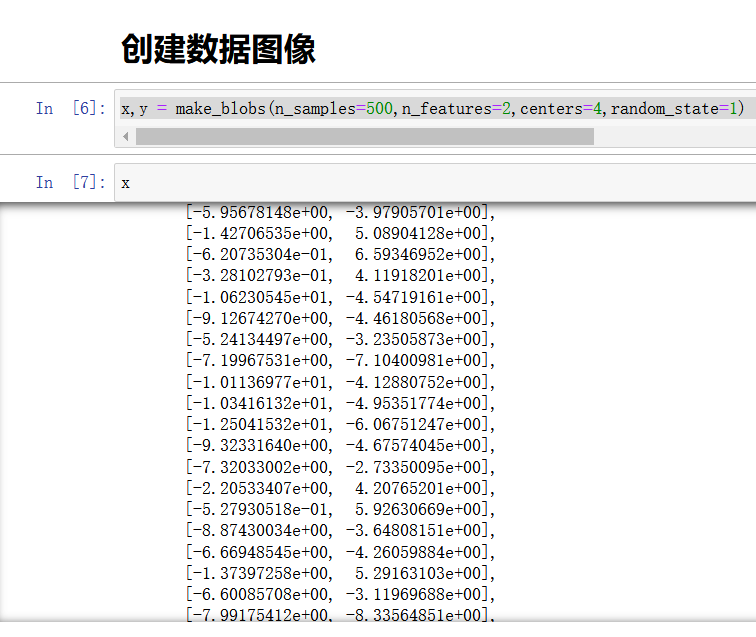在进行聚类之前，查看数据

plt.scatter(x[:,0],x[:,1]
,marker = "o"
,s = 8

)
plt.show()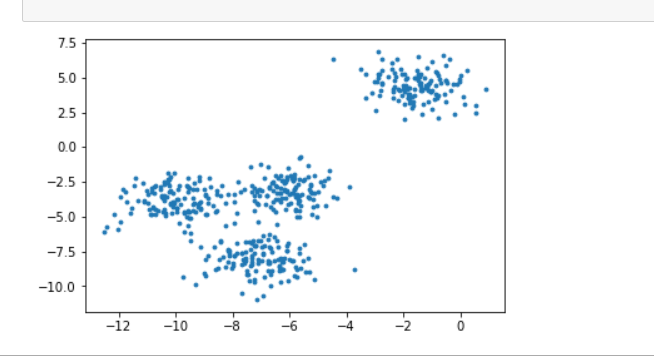from sklearn.cluster import KMeans
cluster = KMeans(n_clusters = 3,random_state=1).fit(x)  #聚类个数为3，实列化+训练
y = cluster.labels_  #查看每个样本的聚类结果
y   #在结果未知下所预测样本结果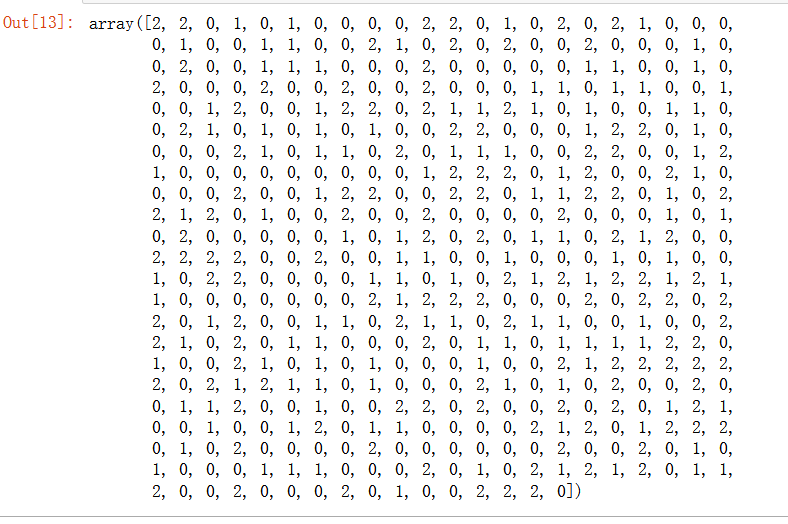聚类模型时间长，所以在大数据进行聚类时，通常使用fit先进行小部分数据聚类，在使用fit_predict对聚类结果进行合并。

cen = cluster.cluster_centers_  #生成3个簇，查看3个质心
cen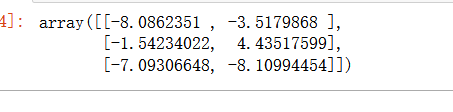可以使用inertia_函数查看簇的距离平方和，但是效果不如轮廓系数。

将聚类结果可视化

color = ["red","pink","orange","gray"]
for i in range(3):           #查看聚类结果
plt.scatter(x[y==i,0],x[y==i,1]
,marker = "o"
,s = 8
,c = color[i]
)

plt.scatter(cen[:,0],cen[:,1]   #查看质心结果
,marker = "o"
,s = 8
,c = "black"
)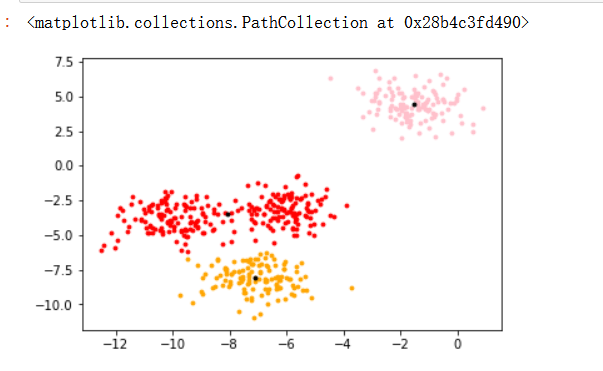使用silhouette轮廓系数进行模型评估

from sklearn.metrics import silhouette_score  #平均轮廓系数
from sklearn.metrics import silhouette_samples #每个样本是轮廓系数
silhouette_score(x,y)  #返回平均轮廓系数 
silhouette_samples(x,y)  #返回每个样本的轮廓系数，求均值为silhoue_score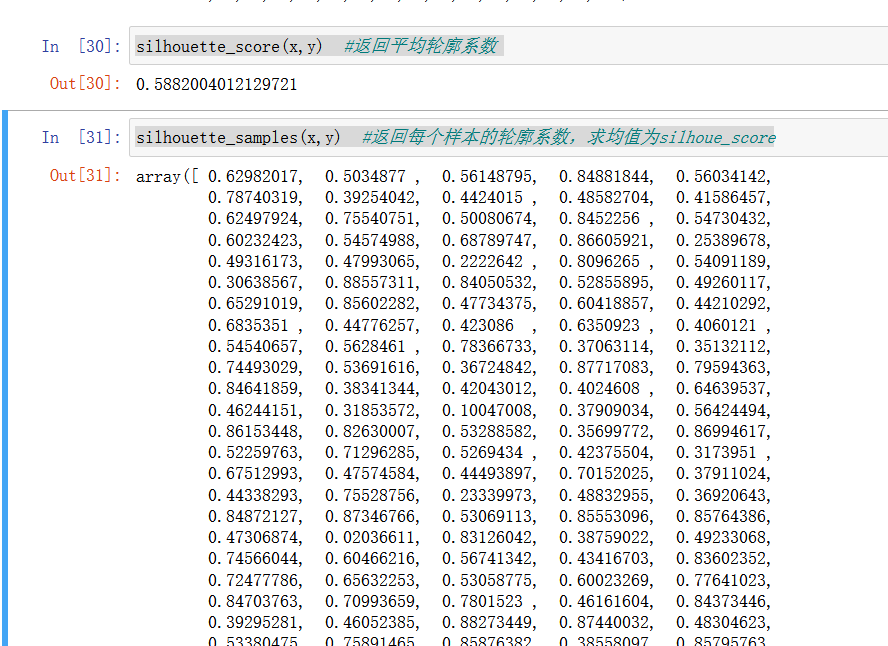也可以使用卡林斯基—哈拉巴斯指数进行评估，优点：速度比轮廓系数快

from sklearn.metrics import calinski_harabasz_score
calinski_harabasz_score(x,y) #评估指数越大越好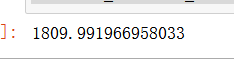展开全文• ## kmeans算法和kmeans++

千次阅读 2021-11-12 21:08:38
kmeans聚类算法 算法原理 kmeans算法原理其实很简单 我用一个最简单的二维散点图来做解释 如上图，我们直观的看到该图可聚成两个分类，我们分别用红点和蓝点表示 下面我们模拟一下Kmeans是怎么对原始的二维散点...

# kmeans算法及其优化改进

## kmeans聚类算法

### 算法原理

kmeans的算法原理其实很简单

我用一个最简单的二维散点图来做解释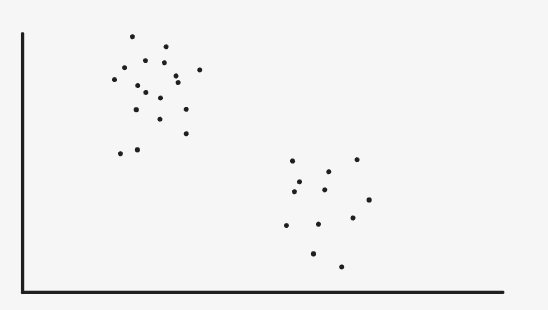如上图，我们直观的看到该图可聚成两个分类，我们分别用红点和蓝点表示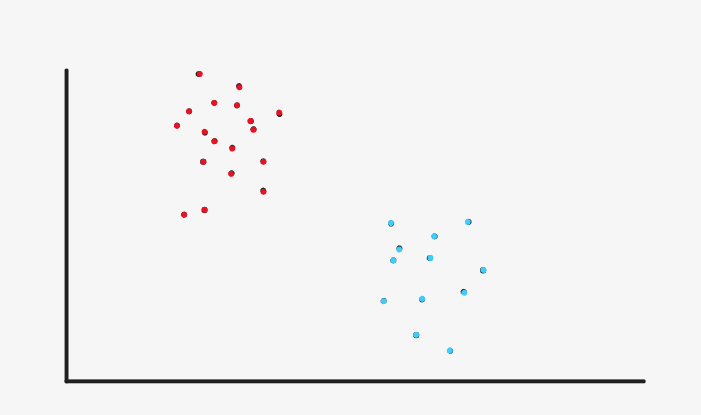下面我们模拟一下Kmeans是怎么对原始的二维散点图做聚类的

首先，随机初始化2个聚类中心（一般是在随机选择两个样本点作为聚类中心）至于什么是聚类中心呢，我们暂且压下不表，现在就把它当成一个点就好。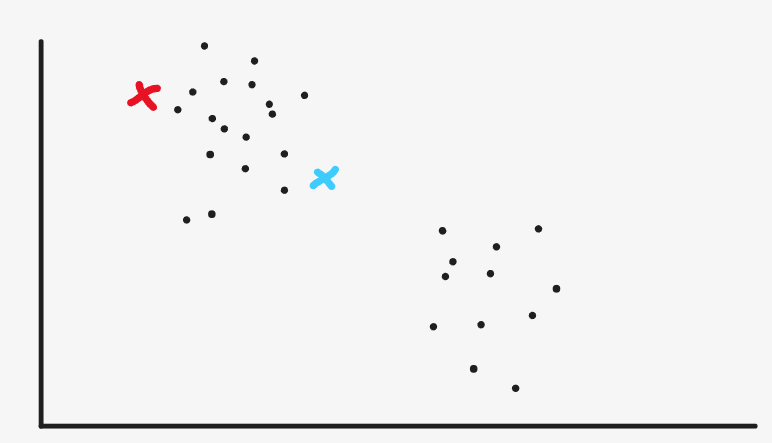然后我们就去把所有离红色点进的样本点标成红色，把离蓝色点近的样本点标成蓝色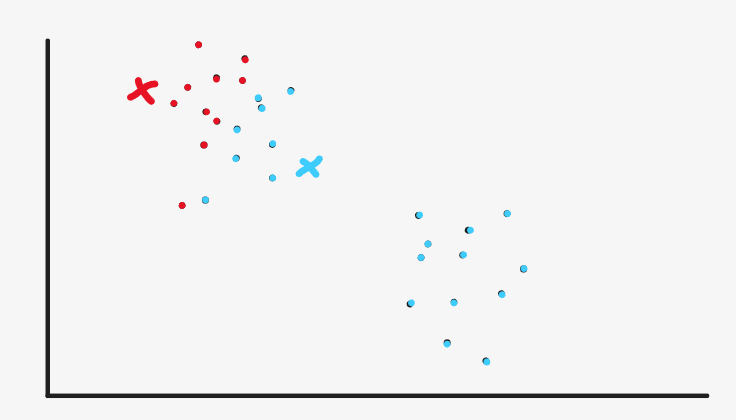然后我们重新设定聚类中心的位置，设在哪呢。红色聚类中心就设在现在的红色点的中心（均值），蓝色聚类中心就设在现在的蓝色点的中心（均值），样本颜色重新设为黑色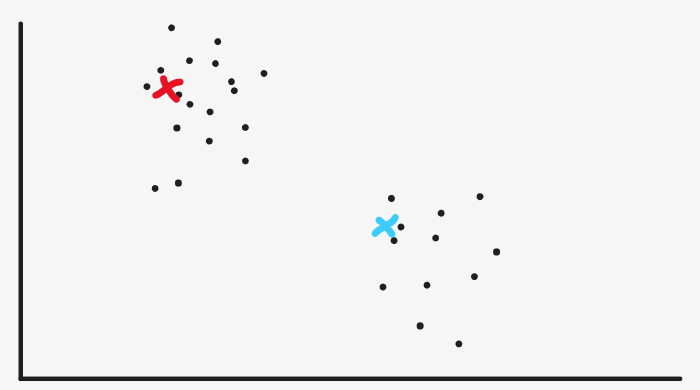然后我们继续把所有离红色点进的样本点标成红色，把离蓝色点近的样本点标成蓝色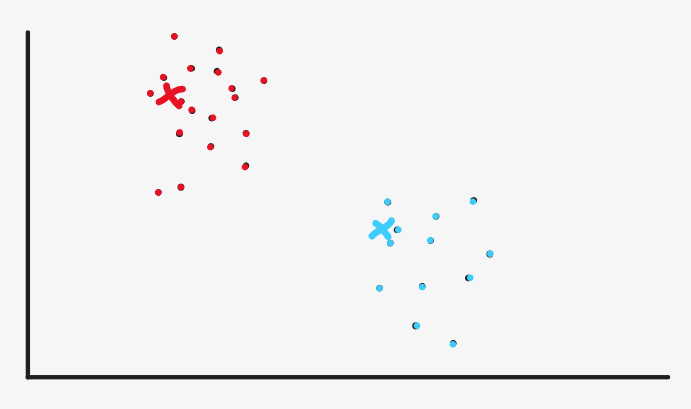其实我们现在看来，红色和蓝色已经很分明了，已经达到最初的效果了，但是机器没有我们的眼睛，机器没办法直观的看到现在已经可以停止了。

所以它会继续按照计算均值的方法重新设定聚类中心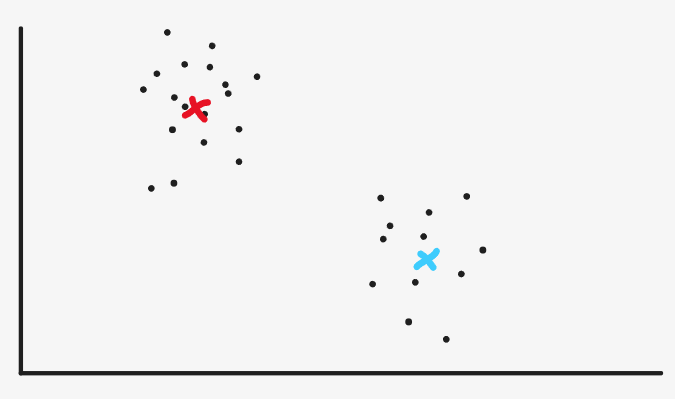然后继续把所有离红色点进的样本点标成红色，把离蓝色点近的样本点标成蓝色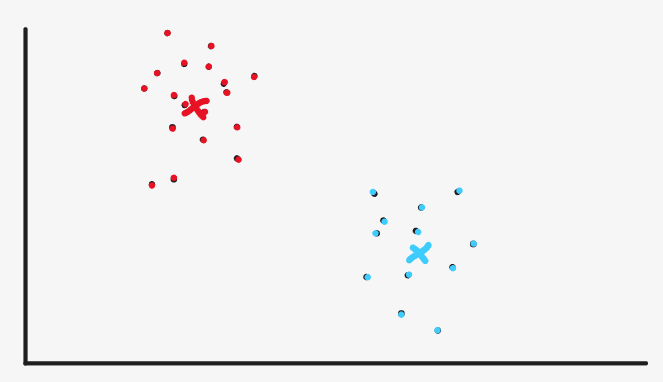然后继续按照计算均值的方法重新设定聚类中心，但到这里机器会发现我们设定的聚类中心和之前的聚类中心的位置几乎没有改变，这说明我们的算法收敛了，每个样本的类别基本已经确定了。于是算法终止，聚类完成。其实在这个地方有两个指标来表示是否终止，一是计算聚类中心位置的变化，二是计算样本聚类的变化，二者是等价的。

### 算法步骤

1. 选定K个聚类中心

2. 计算每个样本点到K个聚类中心的距离，将样本分类设定为距离最小的聚类中心对应分类

3. 计算每个分类集合的样本均值，并将其作为新的聚类中心

4. 重复2，3步骤，直到新的聚类中心与原聚类中心的距离小于设定的阈值即可

### 算法实现

导入包

import numpy as np
import matplotlib.pyplot as plt
from sklearn.datasets import make_blobs


定义模型

class Kmeans:
def __init__(self, k, max_iter=300, thresh=1e-5):
self.k = k
self.thresh = thresh
self.max_iter = max_iter

def random_centroid_init(self,X):
# 随机选取K个样本作为聚类中心
return X[np.random.choice(X.shape, size=self.k)]

def dist(self, x):
return [np.linalg.norm(x - c) for c in self.centroids]

def fit_predict(self, X):
# 初始化聚类中心
self.centroids = self.random_centroid_init(X)
for _ in range(self.max_iter):
# 涂色
y_pred = np.array([np.argmin(self.dist(x)) for x in X])

# 计算新的聚类中心
new_centroids = self.centroids.copy()
for i in range(self.k):
new_centroids[i] = np.mean(X[y_pred==i],axis=0)

# 如果聚类中心位置基本没有变化，那么终止
if np.max(np.abs(new_centroids - self.centroids)) < self.thresh:
break

# 否则更新聚类中心，重复上述步骤
self.centroids = new_centroids
return y_pred


生成数据集

X, y = make_blobs(n_samples=1000, n_features=2, centers=3)


训练

model = KMeans(3, 1e-2)
y_pred = model.fit_predict(X)


可视化结果

plt.figure()
plt.subplot(121)
plt.scatter(X[:, 0], X[:, 1], c=y)
plt.subplot(122)
plt.scatter(X[:, 0], X[:, 1], c=y_pred)
plt.show()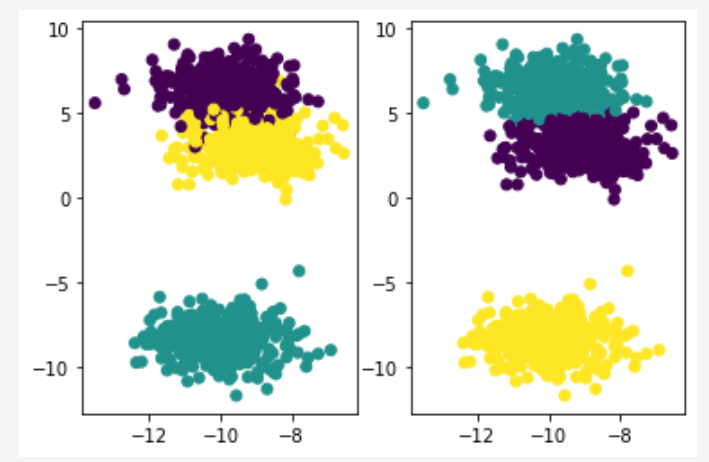## 优化改进

好讲完Kmeans算法，我们再想一想Kmeans算法有什么问题.

### 聚类中心初始化

看下面两张图，它是上面的程序偶尔可能运行出来的结果，左图是运气不好的错误聚类，右边是运气好的正确聚类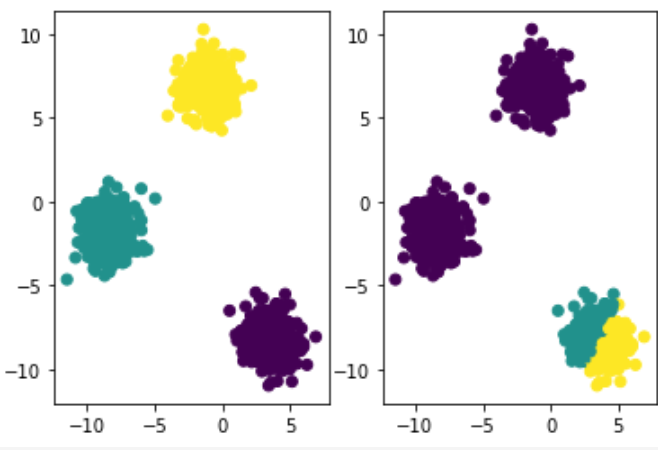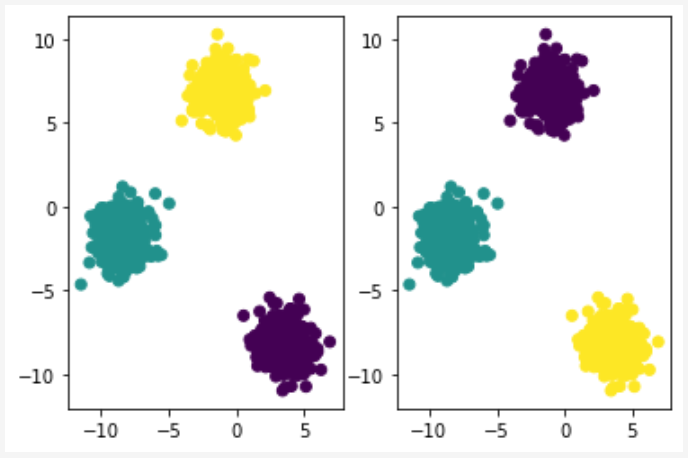程序的两次运行唯一的区别就是聚类中心初始化是随机的，那么现在问题就出在这里

再看我们前面介绍的例子，假如我们的聚类中心是在这个地方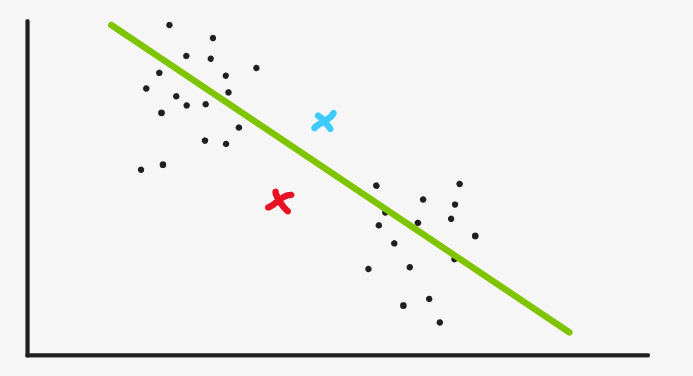于是，绿线下方都被分到红色，绿线上方都分到蓝色，我们一求均值，重新设定聚类中心，发现位置也没有太大改变，于是我们得到的聚类就是这样的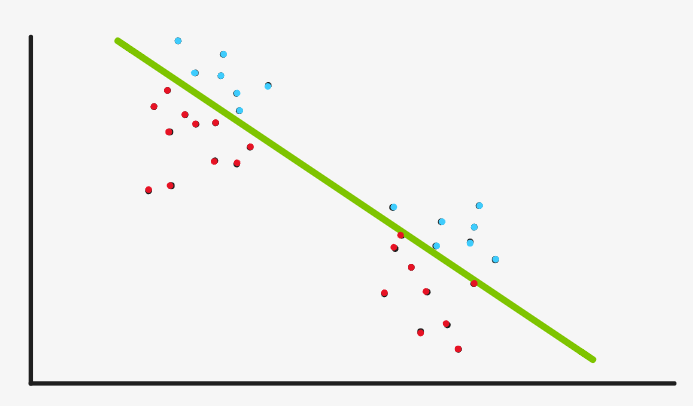很分明，但是显然不是我们希望的聚类

那么这个问题怎么解决，也就是说，随机初始化聚类中心是不好的，我们应该怎样初始化聚类中心？

### Kmeans++算法

为了解决初始化的问题，Kmeans++算法有这样的策略

第一，初始的聚类中心一定是在样本中选，这个与Kmeans算法不同，尽管kmeans算法我们一般也是这样做的，但在算法中并没有对其有实际的限制。而Kmeans++的算法流程中，只有在样本中选才能进行这个算法

第二，选择的K个聚类中心需要尽可能的远。

#### 算法过程

首先随机选取第一个聚类中心，计算各个样本点到距离最近的聚类中心的距离。

让距离越远的点被选择为新的聚类中心的概率越大，重复上述步骤，直到选择出所有的聚类中心。

#### 算法实现

这里怎么将距离的大小体现在选择的概率上其实有很多方式，最极端的一种就是，距离最大的概率为1，其余为0。

实现如下

def max_centroid_init(self,X):
centroids = []
centroids.append(X[np.random.choice(X.shape)])
for i in range(self.k-1):
index = np.argmax([np.min([np.linalg.norm(x - c) for c in centroids]) for x in X])
centroids.append(X[index])
return np.array(centroids)


比较温和一点的是这样，以距离/（距离和）作为每个样本被选择的概率

例如现在有3个样本离自己的聚类中心的距离分别为 5 , 10 , 10 5,10,10 ​，其和为 25 25

我们随机一个 0 0 25 25 之间的数 n u m b e r number ​​​​

如果这个数在 0 0 ​到 5 5 ​以内，我们选择第一个样本，在 5 5 15 15 ​​以内我们选择第二个样本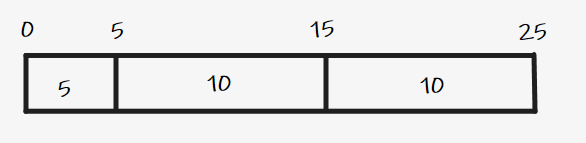其实就是从第一个样本开始遍历， n u m b e r = n u m b e r − D ( x ) number = number-D(x) ​​， n u m b e r number ​​ 什么时候小于0，此时遍历到的样本就是新的聚类中心​

实现如下

def soft_centroid_init(self,X):
centroids = []
centroids.append(X[np.random.choice(X.shape)])
for i in range(self.k-1):
D = [np.min([np.linalg.norm(x - c) for c in centroids]) for x in X]
number = np.random.choice(np.sum(D))
for i,d in enumerate(D):
number -= d
if number<0:
centroids.append(X[i])
break
return np.array(centroids)


其它的改进优化我就不介绍了，我暂时不关注性能问题

### 改进全部代码

import numpy as np
import matplotlib.pyplot as plt
from sklearn.datasets import make_blobs

class Kmeans:
def __init__(self, k, init='pp-soft', max_iter=300, thresh=1e-5):
self.k = k
self.thresh = thresh
self.max_iter = max_iter
self.init = init

def random_centroid_init(self,X):
# 随机选取K个样本作为聚类中心
return X[np.random.choice(X.shape, size=self.k)]

def max_centroid_init(self,X):
centroids = []
centroids.append(X[np.random.choice(X.shape)])
for i in range(self.k-1):
index = np.argmax([np.min(self.dist(x)) for x in X])
centroids.append(X[index])
return np.array(centroids)

def soft_centroid_init(self,X):
centroids = []
centroids.append(X[np.random.choice(X.shape)])
for i in range(self.k-1):
D = [np.min(self.dist(x)) for x in X]
number = np.random.choice(int(np.sum(D)))
for i,d in enumerate(D):
number -= d
if number<0:
centroids.append(X[i])
break
return np.array(centroids)

def dist(self, x):
return [np.linalg.norm(x - c) for c in self.centroids]

def fit_predict(self, X):
# 初始化聚类中心
if self.init == 'random':
self.centroids = self.random_centroid_init(X)
elif self.init == 'pp-max':
self.centroids = self.max_centroid_init(X)
else:
self.centroids = self.soft_centroid_init(X)
for _ in range(self.max_iter):
# 涂色
y_pred = np.array([np.argmin(self.dist(x)) for x in X])

# 计算新的聚类中心
new_centroids = self.centroids.copy()
for i in range(self.k):
new_centroids[i] = np.mean(X[y_pred==i],axis=0)

# 如果聚类中心位置基本没有变化，那么终止
if np.max(np.abs(new_centroids - self.centroids)) < self.thresh:
break

# 否则更新聚类中心，重复上述步骤
self.centroids = new_centroids
return y_pred

X, y = make_blobs(n_samples=1000, n_features=2, centers=3)

model = Kmeans(3)
y_pred = model.fit_predict(X)

plt.figure()
plt.subplot(121)
plt.scatter(X[:, 0], X[:, 1], c=y)
plt.subplot(122)
plt.scatter(X[:, 0], X[:, 1], c=y_pred)
plt.show()

展开全文• 使用这个算法，对不同的点按照到中心点的距离进行分类
• 自适应K-均值聚类算法,能够随着聚簇数目的变化而自动调整聚类数，以最合适的聚簇数目来进行数据分类
• 这个算法咧，其实我以前玩过，不过当时是使用sklearn直接调包的。那么今天呢，我们就先来简单复习。 KMeans 就分几步嘛。 随机选择中心点 计算每一个点离中心点的位置，选择最近的一个点（如果这个点理两个以上的...sklearn
• Kmeans聚类算法-手肘法，jupyter notebook 编写，打开可以直接运行，使用iris等5个数据集，机器学习。
• K-Means聚类是最常见的一种聚类算法。在K-Means聚类中，算法试图把观察值分到k个组中，每个组的方差都差不多。分组的数量k是用户设置的一个超参数。具体来讲，K-Means算如下几个步骤： 随机创建k个分组（即...sklearn
• Kmeans是一个非常基础的聚类算法，使用了迭代的思想，关于其原理这里不说了。下面说一下如何在matlab中使用kmeans算法。 创建7个二维的数据点：复制代码 代码如下:x=[randn(3,2)*.4;randn(4,2)*.5+ones(4,1)*[4 4]];...
• 首先说一下分类和聚类的区别： 分类分类其实就是从特定的数据中挖掘模式，做出相对应的判断。例如对班级的学生进性性别的分类，我事先...KMeans算法是聚类中最常用最普遍的一种算法，该算法最大的特点就是简单，易于大数据
• ## kmeans算法

千次阅读 2021-11-02 20:49:37
文章目录1、概述1.1 无监督学习与聚类算法1.2 sklearn中的聚类算法2、KMeans2.1 KMeans是如何工作的？2.2 簇内误差平方和的定义和解惑3、sklearn.cluster.KMeans3.1 重要参数n_clusters3.2 聚类算法的模型评估指标...机器学习
• Kmeans算法基本思想是：首先给出聚类的个数K，然后初始随机给定K个待聚类中心（也叫簇中心），按照最邻近原则把待分类样本点分到各个类，也就是样本点到哪个簇中心的距离最近，这个样本点就属于哪一类。然后按平均法...
• 数据聚类，主要对于数据点进行聚类，输出节点分类情况和节点中心
• kmeans算法，对影像进行聚类分类，matlab语言，模式识别
• 该程序获取图像和所需的分区数，并找到不同类别的均值并提供分类图像（面具）。matlab
• 基于无监督学习，无需训练数据, 使用SIFT算法提取图像特征，再使用KMeans聚类算法进行图像分类。 对源代码进行了优化，实现了对应图片自动分类到各自文件夹功能，并且优化了分类准确率。 设计思路： 1）首先编写...
• 框架：keras，语言：python，使用算法：k-means，需要安装一些安装包
• 本节内容：本节内容是根据上学期所上的模式识别课程的作业整理而来，第一道题目是Kmeans聚类算法，数据集是Iris(鸢尾花的数据集)，分类数k是3，数据维数是4。 关于聚类  聚类算法是这样的一种算法：给定样本数据...
• Python基于KMeans算法进行文本聚类项目实战.zip,data.csv,代码讲解+KMeans文本聚类.mp4,stop_words.utf8,Python基于KMeans算法进行文本聚类项目实战.pdf,nlp_KMeans.py
• 工具：jupyter notebook, 包含文件：ipython源码及excel数据集。使用Kmeans模型进行用户分群，肘部法则判断聚类个数，根据概率密度图对用户进行分类
• 来了一个样本x，要给它分类，即求出它的y，就从数据集中，在x附近找离它最近的K个数据点，这K个数据点，类别c占的个数最多，就把x的label设为c 区别 1.K-Means是聚类算法2.非监督学习3.喂给它的数据集是无label的...数据挖掘
• ——以二维数组、鸢尾花...聚类算法属于无监督学习，其中的KMeans算法是将一组N个样本的数据划分成K个不相交的 clusters （簇） C。 means （均值）通常被称为 cluster（簇）的 “centroids（质心）”; 注意，它们...聚类算法 python 数据分类
• 分类是指将数据归于一系列已知类别之中的某个类的分类过程分类作为一种监督学习方法要求必须事先明确知道各个类别的信息并且断言所有待分类项都一个类别与之对应但是很多时候上述条件得不到满足尤其是在处理海量...
• 给出了kmeans算法的具体应用实例，可以供做kmeas分类的人员参考！
• 使用Kmeans分类算法，为各国体育水平分类。 体育竞赛数据使用1988年至2012年奥运会奖牌数据 一枚金牌得5分 一枚银牌得3分 一枚铜牌得1分 德国和俄罗斯比较特殊，东西德统一前，他们各自参赛，终究是一个民族，因此将...Kmeans分类...